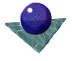Expressing Pose:
rotation and translation matrices and vectorsFigure 2, below, illustrates the basic problem. There are two matching point sets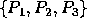or, alternatively, a matching point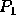and two vectors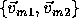in the model which match two vectors in the scene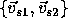. There are several different transformations which superimpose the model set on the scene set (or vice versa).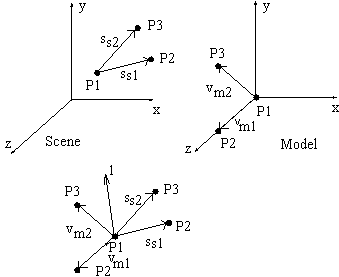Figure 2: Transformation of 3 points, or a point-vector-vector
With reference to Equation 3,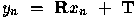, the basic rotation matrix is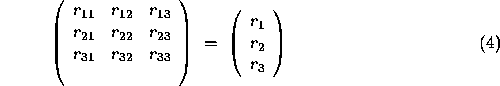and the basic translation matrix isThese can be combined to formrepresenting the point to be transformed as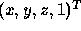in homogeneous coordinates. If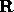is a rotation matrix in 3D orthogonal space, then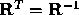and the determinant ofis 1. Representing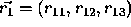and so on this gives 6 constraint equations,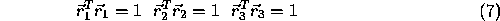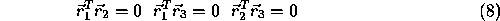The first intuitive approach to define a rotation matrix might be the fixed axis method , e.g.

• Translate pointto the origin
• Rotate byabout the original x-axis (positive rotation is from y to z)
• Rotate by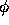about the original y-axis (positive rotation is from z to x)
• Rotate by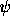about the original z-axis (positive rotation is from x to y)

This leads to a rotation matrix formed by the concatenation of the matrices for the three single angle rotations about the fixed axes,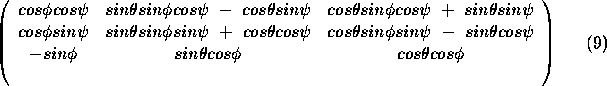Note also that the angles can be recovered,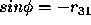,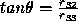and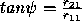.

A second method to define a rotation matrix, illustrated in Figure 2, above, is based on a rotation about an arbitrary axis,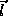, by an angle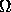.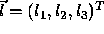,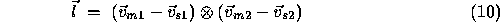andis defined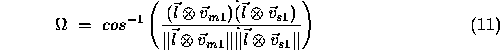The rotation matrix is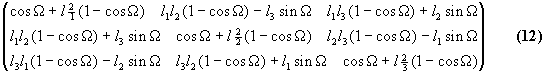Re-arranging, we can also expressandin terms of the rotation matrix elements.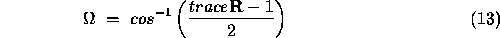The trace of a square matrix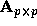is defined as the sum of the diagonal elements,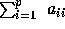. N is a normalisation operator,.Comments to: Sarah Price at ICBL.